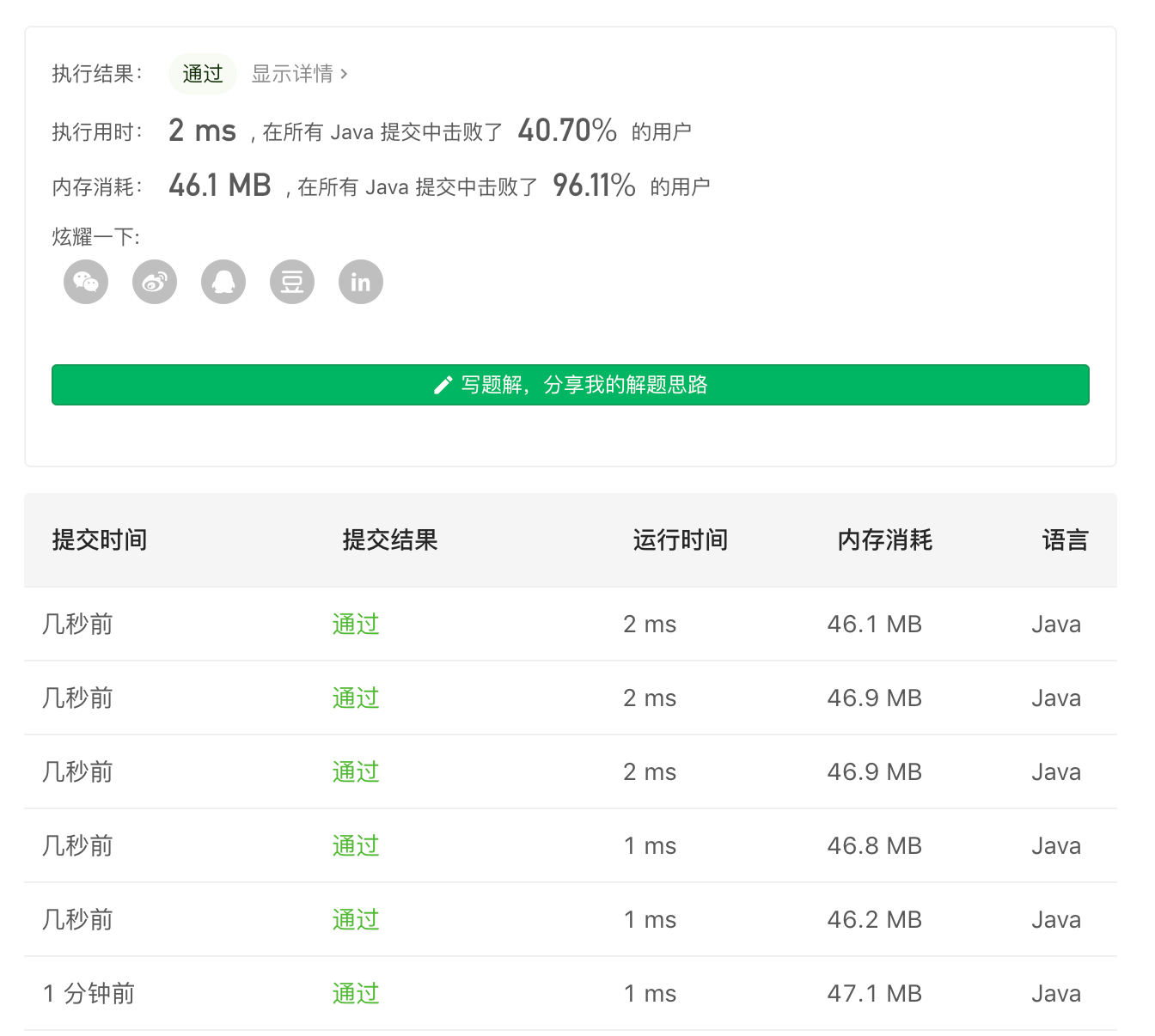# 「两次遍历」要比「一次遍历」要慢 ... 为啥呀？为啥呀？？## 题目描述

• 如果对于所有 i <= j，A[i] <= A[j]，那么数组 A 是单调递增的。

• 如果对于所有 i <= j，A[i]> = A[j]，那么数组 A 是单调递减的。

输入：[1,2,2,3]输出：true

输入：[6,5,4,4]输出：true

输入：[1,3,2]输出：false

输入：[1,2,4,5]输出：true

输入：[1,1,1]输出：true

• 1 <= A.length <= 50000

• -100000 <= A[i] <= 100000

## 朴素解法（所谓的两次遍历）

class Solution {    public boolean isMonotonic(int[] a) {        return check(a, true) || check(a, false);    }    boolean check(int[] a, boolean flag) {        for (int i = 0; i < a.length - 1; i++) {            if (flag) {                if (a[i] > a[i + 1]) return false;            } else {                if (a[i] < a[i + 1]) return false;            }        }        return true;    }}

• 时间复杂度：$O(n)$

• 空间复杂度：$O(1)$

## 朴素解法（所谓的一次遍历）

class Solution {    public boolean isMonotonic(int[] a) {        boolean up = true, down = true;        for (int i = 0; i < a.length - 1; i++) {            if (a[i] > a[i + 1]) up = false;            if (a[i] < a[i + 1]) down = false;            if (!up && !down) return false;        }        return up || down;    }}

• 时间复杂度：$O(n)$

• 空间复杂度：$O(1)$

## 总结## 有趣的实验

class Solution {    // 统计「二次循环」的访问次数    int cnt;    public boolean isMonotonic(int[] a) {        cnt = 0;        // 这里不直接写成「短路与」进行返回，确保两个循环都会被执行        boolean t = check(a, true), u = check(a, false);         System.out.println(cnt);        return t || u;    }    boolean check(int[] a, boolean flag) {        for (int i = 0; i < a.length - 1; i++) {            if (flag) {                if (getVal(a, i) > getVal(a, i + 1)) return false;            } else {                if (getVal(a, i) < getVal(a, i + 1)) return false;            }        }        return true;    }    int getVal(int[] a, int idx) {        cnt++;        return a[idx];    }}

class Solution {    // 统计「一次循环」的访问次数    int cnt;    public boolean isMonotonic(int[] a) {        cnt = 0;        boolean up = true, down = true;        for (int i = 0; i < a.length - 1; i++) {            if (getVal(a, i) > getVal(a, i + 1)) up = false;            if (getVal(a, i) < getVal(a, i + 1)) down = false;            if (!up && !down) break;        }        System.out.println(cnt);        return up || down;    }    int getVal(int[] a, int idx) {        cnt++;        return a[idx];    }}

## 最后## 评论